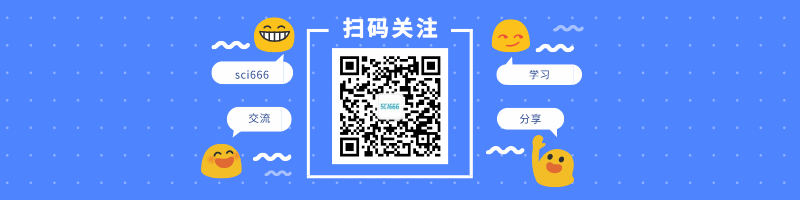# CIBERSORT肿瘤免疫微环境分析教程

`````` 1# boxplot
2ggboxplot(
3  plot.info,
4  x = "CellType",
5  y = "Composition",
6  color = "black",
7  fill = "CellType",
8  xlab = "",
9  ylab = "Cell composition",
10  main = "TME Cell composition"
11) +
12  theme_base() +
13  theme(axis.text.x = element_text(
14    angle = 90,
15    hjust = 1,
16    vjust = 1
17  ))
```````````` 1# box plot group by GRADE
2ggboxplot(
3  plot.info,
4  x = "CellType",
5  y = "Composition",
6  color = "black",
8  xlab = "",
9  ylab = "Cell composition",
10  main = "TME Cell composition group by GRADE"
11) +
12  stat_compare_means(
13    label = "p.signif",
14    method = "t.test",
15    ref.group = ".all.",
16    hide.ns = T
17  ) +
18  theme_base() +
19  theme(axis.text.x = element_text(
20    angle = 90,
21    hjust = 1,
22    vjust = 1
23  ))
```````````` 1# Barplot of cell componment of each sample using hclust
2sample.index <-
3  hclust(dist(TME.data[, TME.cells]), method = "ward.D")\$order
4sample.order <- TME.data\$PATIENT_ID[sample.index]
5
6ggbarplot(
7  plot.info,
8  x = "PATIENT_ID",
9  y = "Composition",
10  size = 0,
11  fill = "CellType",
12  color = "CellType",
13  order = sample.order
14) +
15  theme_base() +
16  theme(
17    axis.text.x = element_text(
18      angle = 90,
19      hjust = 1,
20      vjust = 1,
21      size = 1
22    ),
23    legend.position = "bottom"
24  )
``````## 利用python爬取并翻译GEO数据库

2020-4-27 21:10:00

## 10X Genomics V(D)J测序:免疫分析从零起步学(1)

2020-4-28 15:27:20

Notice: When your legal rights are being violated, please send an email to: [email protected]3 条回复 A文章作者 M管理员
1. 同问，数据格式是如何，或者请问有详细的连贯教程吗

2. 请问plot.info的数据格式是怎样的，有小伙伴解决这个问题了吗

3. 感谢分享，可以请求分享一下plot.info的数据吗？我基础差，实在画不出来